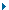Institut Camille Jordan - UMR 5208 Université Claude Bernard Lyon 1 43 boulevard du 11 novembre 1918 F - 69622 Villeurbanne cédex tel : (33) 04 72 44 81 13 email : click here Francis Clarke Mathematician professeur titulaire, Université Claude Bernard Lyon 1

Research interests :Nonsmooth and functional analysisControl theoryCalculus of variationsDifferential equationsOptimizationMathematical modelingNew Book:Functional Analysis, Calculus of Variations and Optimal Control, Graduate Texts in Mathematics Vol. 264, Springer, 2013. (For details and weblink, click here: ) ( pdf)Corrections to the book "Functional Analysis, Calculus of Variations and Optimal Control"
( pdf)Short biography
( pdf)Publication list
( pdf)Errata to Nonsmooth Analysis and Control Theory, by F. Clarke, Yu. Ledyaev, R. Stern and P. Wolenski, Graduate Texts in Mathematics Vol. 178, Springer-Verlag, New York, 1998
(pdf)Discontinuous Feedback and Nonlinear Systems (Tutorial, IFAC NOLCOS Bologna 2010)
( pdf)Optimal Control Problems with Mixed Constraints (w. MdR de Pinho). SIAM J Control Optim. 48 (2010) 4500-4524.
( pdf)An Extension of the Schwarzkopf Multiplier Rules (w.Yu. Ledyaev and MdR de Pinho . SIAM J. Control Optim. 2011.
( pdf)Stability analysis of sliding-mode feedback control (w. R. B. Vinter) Control and Cybernetics 38 (2009) 1151-1167.)
( pdf)Regularity of solutions to one-dimensional and multi-dimensional problems in the calculus of variations, Proceedings of the Rome conference, June 2006.
( pdf)Nonsmooth Analysis in Systems and Control Theory, Encyclopedia of Complexity and Systems Science.
( pdf)A Lipschitz regularity theorem, Ergod. Th. & Dynam. Sys.27 (2007 )1-6.
( pdf)A maximum principle for hybrid optimal control problems, CDC2006.
( pdf)Necessary conditions in optimal control and in the calculus of variations, Proceedings of the Aveiro conference, to appear.
( pdf)Necessary Conditions in Dynamic Optimization, Memoirs of the Amer. Math. Soc., No. 816, vol. 173 (2005) (113 pages).
(dvi, pdf)Continuity of solutions to a basic problem in the calculus of variations, Annali Scuola Normale Superiore Pisa (Serie V) 4 (2005) 511-530.
(dvi, pdf)Local Lipschitz continuity of solutions to a problem in the calculus of variations, with Pierre Bousquet,J. Diff. Equations 243 (2007) 489-503.
(ps, pdf)Lyapunov functions and feedback in nonlinear control, in "Optimal Control, Stabilization, and Nonsmooth Analysis", M.S. de Queiroz, M. Malisoff and P. Wolenski (Eds.), Lecture Notes in Control and Information Sciences 301, pp. 267-282, Springer-Verlag 2004.
(pdf)The Maximum Principle in Optimal Control, then and now, J. Control and Cybernetics 34 (2005) 709-722.
(dvi, pdf)Lyapunov and feedback characterizations of state constrained controllability and stabilization, with R. Stern, Systems & Control Letters 54 (2005) 747-752.
(dvi, pdf)Nonsmooth analysis in control theory: a survey, European J. Control 7 (2001) 63-78.
( pdf)Feedback stabilization and Lyapunov functions, with Yu. Ledyaev, L. Rifford and R. Stern, SIAM J. Control Optim. 39 (2000) 25-48.
( dvi)